# Polish Notation in Data Structure

In this article, we will look into Polish notation in Data Structures. We will discuss its types along with some examples and the use of such notations in general.

Polish Notation is a general form of expressing mathematical, logical and algebraic equations. The compiler uses this notation in order to evaluate mathematical expressions depending on the order of operations. There are in general three types of Notations used while parsing Mathematical expressions:

• Infix Notation
• Prefix Notation
• Postfix Notation

Infix Notation or Expression is where the operators are written in between every pair of operands. It is the usual way to write an expression generally written with parentheses. For Ex: An expression like X+Y is an Infix Expression, where + is an Operator and X, Y are Operands.

## Polish Notation in Data Structure

Now, Polish Notation is also known as Prefix Notation or Expression. In this type of arithmetic expression, the operators precede the operands i.e. the operators are written before the Operands. The operators are placed left for every pair of operands. So, for the above Infix X+Y, its equivalent Polish or Prefix Notation is +XY.

### Evaluation with Example

Now, let us look at an example on how to evaluate a Polish Notation or Prefix Expression to get the result.

Consider this Expression : / * + 5 6 3 11. While evaluating the expression we take decision for two cases: When the Character is an Operand or When the Character is an Operator. Let us look at the steps.

Step 1:

We will use a Stack for this evaluation.We scan the Expression from right to left, if the current character is an Operand we push it into the stack. So from 11 to 5 we push the elements into the stack. The stack looks: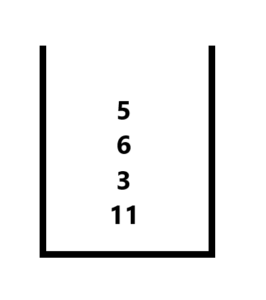Step 2:

As soon as we get an operator we multiply its previous two elements, so continuing traversing from right to left we first get ‘+’ operator so we pop two elements from stack (5 & 6) compute their result with the operator i.e. 5+6 = 11, and push the result back into the stack for future evaluation. The Stack now is: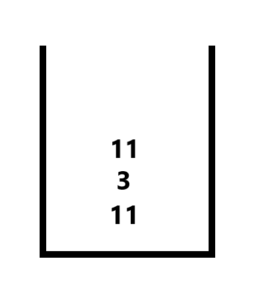Step 3:

The next Operator is ‘*’ Operator (Multiply), so we again pop the two elements from stack and repeating the process of Step 2. So we compute the result from their operation (11 * 3 =33) and push it back to the stack again. The stacks now look like: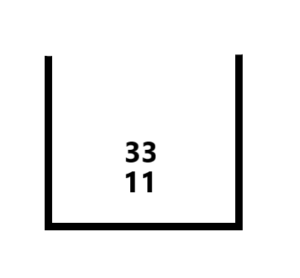Step 4:

Finally, we have the ‘/’ operator so we pop 33 and 11 compute the result push it back to the stack. Now we have reached the leftmost or start index of the expression so at this point our stack will contains only one value which will be our Resultant Evaluated Prefix Expression.

Let us look at the implementation code for this in Java:

Output:

## Reverse Polish Notation

Now, Polish Notation has Another Type – Reverse Polish Notation or also known as Postfix Expression. These are the expression where the Operands precede the Operators i.e. the Operands are written before the Operators. For Example: The Infix X+Y will be represented in Postfix or Reverse Polish as XY+.

### Evaluation with Example

Now, let us see how to evaluate a given Postfix Expression. Consider this Reverse Polish or Postfix Expression: 4 3 2 + * 5 – .

Step 1:

We will again use a Stack for this evaluation. Here, We scan the Expression from left to right, if the current character is an Operand we push it into the stack. So from 4 to 2 we push the elements into the stack. The stack looks: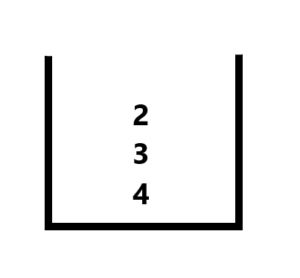Step 2

Now, on traversing next we get ‘+’ operator, so we pop two elements from the stack compute their result and push it back again for future evaluation. The steps here are same as above discussed example. The difference is that in this case we traverse from left to right. The overall algorithm remains same. The stack now is: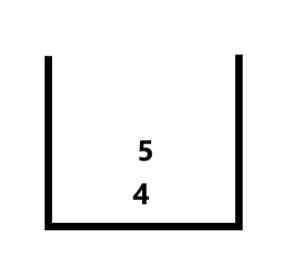Step 3:

Now, computing all the steps for each operator we get ‘*’ so we pop 5 and 4 and push 5 * 4 = 20 into stack and then we get 5 so we push into stack then finally we get ‘-‘ operator so we compute their result 5-20 = -15, then we push it again, at the end index of the string we get the result of our Postfix evaluation. The stack finally has -15.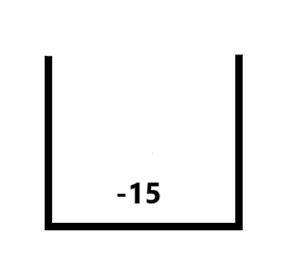Let us look at the implementation code in Java:

Output:

### Polish Notation Applications

• Polish Notation is useful in representing the Mathematical Expression for the machines to understand them.
• The compiler can easily evaluate these expressions without having to scan the expression for operators first then for operand which requires multiple scanning. By converting the Infix expression to Polish notation the compiler can then evaluate the expression in one go.
• The modern Stack-organized computers are better suited for postfix and prefix notation than the traditional infix notation.

So that’s it for the article you can try out the above discussed steps with different examples and execute the code for better understanding. Feel free to leave your suggestion or doubts in the comment section below.